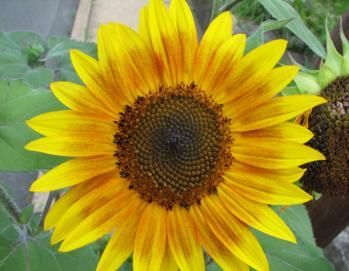Home Practice
For learners and parents For teachers and schools
Textbooks
Full catalogue
Pricing SupportLog in

We think you are located in United States. Is this correct?

# Chapter 3: Number patterns

• This chapter covers investigating number patterns that involve a common difference and the general term is linear.
• Arithmetic sequences are only covered in grade 12 so do not use $$T_{n} = a + (n - 1)d$$ here.
• The focus of this chapter is more about investigating patterns in numbers and diagrams rather than on formulae.

## 3.1 Introduction (EMAX)

In earlier grades you saw patterns in the form of pictures and numbers. In this chapter, we learn more about the mathematics of patterns. Patterns are repetitive sequences and can be found in nature, shapes, events, sets of numbers and almost everywhere you care to look. For example, seeds in a sunflower, snowflakes, geometric designs on quilts or tiles, or the number sequence $$0; 4; 8; 12; 16; \ldots$$.The pattern of seeds within a sunflower follows the Fibonacci sequence, or $$1; 2; 3; 5; 8; 13; 21; 34; 55; 89; 144; \ldots$$

Interested in learning more about the relationship between Fibonacci sequences and sunflowers?

Video: 2F6Y

Try spot any patterns in the following sequences on your own:

1. $$2; 4; 6; 8; 10;\ldots$$

2. $$1; 2; 4; 7; 11; \ldots$$

3. $$1; 4; 9; 16; 25; \ldots$$

4. $$5; 10; 20; 40; 80; \ldots$$

temp text

Identifying patterns in sequences.

Video: 2F6Z